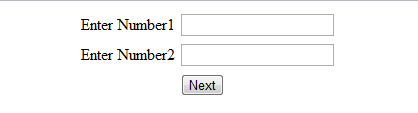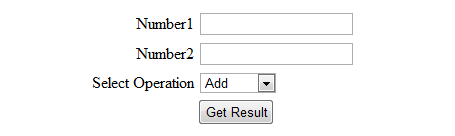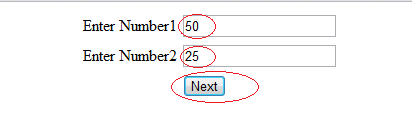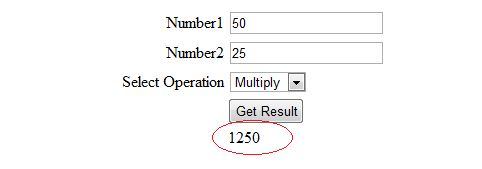We use QueryString property of Request Object for passing variables content between pages ASP.NET. QueryStrings are data that is appended to the end of a page URL. They are commonly used to hold data like page numbers or search terms or other data that isn't confidential.

##### Example

Here is the example which shows you to Pass the values in QueryString and further retrieve it.

1.      Create a page named Default.aspx and design the following UI.On Next Button Click Event Handler write the following code

`protected void btnNext_Click(object sender, EventArgs e)    {        // it will Pass the value and call the Result.aspx page        Response.Redirect("Result.aspx?Num1=" +txtNum1.Text + "&Num2=" +txtNum2.Text);    }`

2.      Now Create another page names Result.aspx and design the following UI.3.      Write the following code on Page_Load() of Result.aspx

`string operation = "";    double number1,number2,result;    protected void Page_Load(object sender, EventArgs e)    {               if (Page.IsPostBack== false)        {            //Getting the value fromQueryString            txtNum1.Text= Request.QueryString["Num1"];            txtNum2.Text= Request.QueryString["Num2"];                   }           }`

4.      On Get Result Button Click Event Handler write the following code

` protected void btnCalculate_Click(object sender, EventArgs e)    {        number1 = Convert.ToDouble(Request.QueryString["Num1"]);        number2 = Convert.ToDouble(Request.QueryString["Num2"]);        operation = cbOperation.SelectedValue.ToString();        switch (operation)        {            case "Add":                result = number1 + number2;                break;            case "Subtract":                result = number1 - number2;                break;            case "Multiply":                result = number1 * number2;                break;            case "Divide":                result = number1 / number2;                break;            default:                result = 0;                break;        }        lblResult.Text = result.ToString();    }`

5.      Now, focus to Default.aspx page and run the Website.

6.      Give the Required value and then Click Next Button.

7.      On Next Page, Value in the textboxes will be filled automatically with the help of value passed in QueryString from Default.aspx page.

8.      Choose the desired operation and Click Get Result Button to Get the result of the calculation.

##### Output Window 1.0##### Output Window 1.1Modified On Nov-30-2017 01:16:56 AM